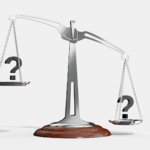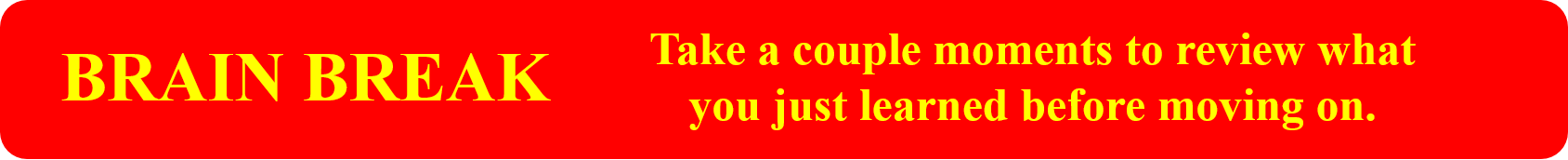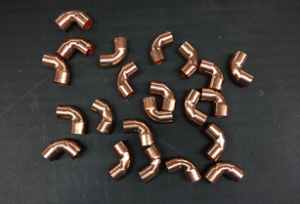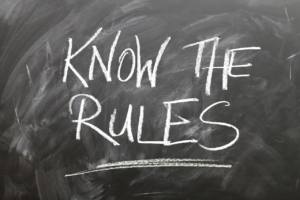Decimals

# Compare DecimalsChad wants to make sure that all his students understand the value of money, and an important aspect of that is knowing how to accurately determine the sizes of decimals. Take a look at the following four decimals.

1. 8.16
2. 8.151
3. 8.1513
4. 8.15138

Which of these is the largest? To answer the question, you would have to compare decimals.

At first glance, you might say the largest number is 8.15138, as it has the most digits and may look like the largest. But if we referred back to the chapter on Understanding Decimal Notation, we would remember that, as you go further to the right from the decimal point (.), the numbers become smaller. So in the example 8.15138, the 3 near the end is worth far less than the 5 two spots to the left of the decimal point.If we followed this logic, then the largest number of the four would be 8.16, even though it has the fewest digits. When comparing decimals, we really have to start with the whole number. In this situation, it’s 8 in all four answers, so it doesn’t come into play.

Once we have decided that the whole number is equal in all four cases, the next thing we have to look at is the number directly to the right of the decimal. This is the tenths place and is worth the most in decimals. Once again, all four answers give us a value of 1 in the tenths column, so this will not be the determining factor. Move one space to the right again and you get the hundredths place. All the numbers contain a 5, except the first number, where we have a 6. Therefore, this is the defining place value.I’ll go through another example, and then you can try a couple on your own.

Example

Which of the following is the largest number?

1. 7.34
2. 7.332
3. 7.323
4. 7.3234

Following the same pattern as before, we would go through the following steps.

Step 1: Start with the whole number. Which number has the largest whole number? As they are all equal, this will not be the determining factor.

The whole number in all four cases is a 7. This is not the deciding factor.

Step 2: Go to the first digit to the right of the decimal. It works out that the digit is a 3 in all cases. Therefore, this is not the deciding factor, either.

The number in the tenths column in all four cases is a 3. This is not the deciding factor.

Step 3: Go one place to the right again. This would be the hundredths place. Here, we find the first difference. Answer a gives us the largest number in this column. Therefore, answer a is the largest number.

The digit in the hundredths column is different for each number. In this case, the number with the highest value in the hundredths column is the largest number.

Therefore, the largest number of the four is:

$\LARGE7.34$

# Practice Questions

Now try a couple for yourself and check out the video answers.

Find the largest number.

Question 1

1. 27.5
2. 27.54
3. 27.539
4. 27.5392

Question 2

1. 14.001
2. 13.099
3. 13.999
4. 13.001# Rounding DecimalsDuring the course, Chad talks about the importance of money and how to work effectively with it, especially when it comes to bookkeeping. Making sure that you track business transactions down to the cent (or decimal). As we have 100 cents in a dollar, if we were to round accounting totals, we would be rounding to the nearest 10 cents (tenths) or even to the nearest dollar (ones).

Before we get to rounding decimals, we should start off with rounding in general. The students are asked to count fittings on a table.How many did you count?

There are a total of 22 fittings on the table, and the students are now asked to round the number of fittings to the nearest 10. This would mean rounding up or down to 10, 20, 30, 40, and so on. This example may seem straightforward, as we are much closer to 20 than to 30, and if you answered 20, then you would be correct. But what is the rule that we follow?RULE: Find the place value we are rounding (in this case, the tens position). Look at the digit to the right of that number (in this case, the ones place). If that number is 4 or less, we round down. If that number is 5 or greater, we round up.

In our example with the 22 fittings, we have a 2 in the ones column, so we end up rounding down to 20. If the number in the ones column were 5 or greater, we would round up to 30.Let’s go through a couple of quick example questions before we move on.

Round the following numbers to the nearest 10. Remember your place value system here.

## Questions1. 12
2. 27
3. 45
4. 98

1. 12 rounds down to 10
2. 27 rounds up to 30
3. 45 rounds up to 50
4. 98 rounds up to 100

Check out the answer for d. When rounding the tens column, we end up rounding up. We run out of room in the tens column, as the highest round number we can make with it is 90. This forces us to move into the hundreds column and start with 1, making the answer 100.

Now that we have rounding whole numbers solved, let’s move onto rounding decimals. Rounding decimals follows the same principles. It’s important here to remember the place value system, not only for whole numbers, but also for decimals. Note the following rule when dealing with numbers:As you go from right to left in a number, including the decimals, the value of the digits becomes greater. When dealing with decimals, this principle means that, as you go further to the right from the decimal, you are dealing with smaller and smaller portions of a whole number.

Example

Round the following number to the nearest hundredth.

$\LARGE123.45678$

Step 1: Identify which number is in the hundredths column.Step 2: Look at the number directly to the right of the hundredths column. In this case, it would be the digit in the thousandths column, which is 6.

Step 3: Follow the rules of rounding.

RULE: If the number is 4 or less, round down. If the number is 5 or greater, round up.

As we have 6 in the thousandths column, we round up.

Therefore, 123.4567 rounded to the nearest hundredth is:

$\LARGE123.46$

# Practice Questions

Round the following decimals. Make sure to heed the place value system when answering the questions. Check the video answers to see if you are correct.

Question 1

Round to the nearest tenth.

$\LARGE27.71536$

Question 2

Round to the nearest thousandth.

$\LARGE14.75638$

Question 3

Round to the nearest hundredth. This is a new concept. Before checking the video answer, give this one a try and see how you do.

$\LARGE75.9999$